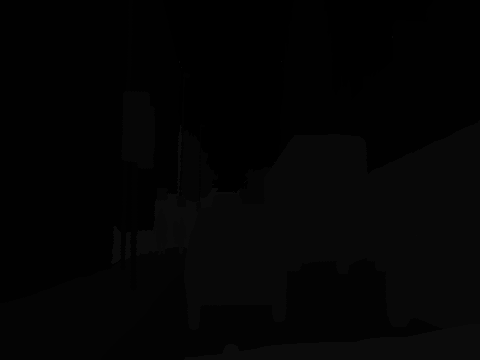# Can unet class output a masked image, just the the black and grey one?

Can unet class output a masked image, just the the black and grey one?
If so, how?

Are you using v2?

nope~~~~ you are not going to tell me v1 do not do that.

Ow, of course you can do that in v1, I just don’t know how lol

do you know how to convert tensor to PIL image?

You’d need to mix up the channels a bit, first few google searches came up with some decent options. https://discuss.pytorch.org/t/pytorch-pil-to-tensor-and-vice-versa/6312/3

And are you asking if unet can output a 2 channel image?

or are you talking about binary segmentation ?
@muellerzr don’t you have a scaling function in the segmentation notebook ? That should work right?

I want to turn back to the black and grey image just like the mask in lesson 3. At pixel x and y, it is a bird…and so on. Thank You if you can help me.Yes, I think we’re talking about saving the mask away as an image, yes @JonathanSum? if so this can convert raw tensor:

``````
for i, pred in enumerate(preds):
pred_arg = pred.argmax(dim=0).numpy()
rescaled = (255.0 / pred_arg.max() * (pred_arg - pred_arg.min())).astype(np.uint8)
im = Image.fromarray(rescaled)
im.save(f'Image_{i}.png')
``````

Assume preds is the result from learn.get_preds

convert tensor array to numpy array using `.numpy()`
convert array to pil image - `Image.fromarray()`
now you could use pil’s `.getcolors()’
you should have the unique colors in the PIL image now.
map these unique color to distinct numbers to form a new image
I think that should work

1 Like